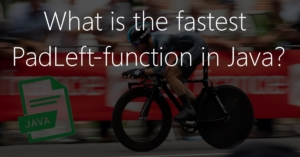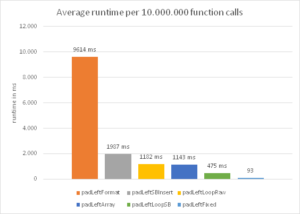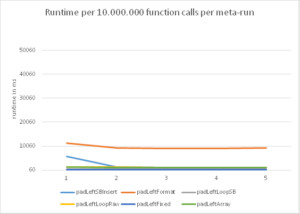Since Java does not have a function to pad strings on the left or right side, you either have to program such function yourself or use an existing library, such as Apache Commons. Because I recently needed a function that would pad a numeric string on the left side (= padLeft) with zeros up to a length of 8 characters, but did not want to include a whole library for such a simple task, the only option was to write a padLeft function myself.

However, when researching and implementing, I found various solution approaches and so I ended up with six different padLeft implementations. In order to find the best padLeft function for my use case, I tested all six implementations during a small performance test and found the fastest padLeft function. Before I get to the results, I would like to briefly describe the individual functions.

## padLeft in Java – six variants

The first version (padLeftFormat) is one of the shortest implementations and works with the format method of the String class. The format method takes the complete work and fills up the string by any given length with 0. Disadvantage: The function can only fill zeros.

```private static String padLeftFormat(String input, int padUpTo){
return String.format("%0" + padUpTo + "d", Integer.parseInt(input));
}
```

The second version (padLeftLoopRaw) is based on a for-loop that runs as many times as it needs to fill characters. The string processing is performed by means of the “+=”-operator. Advantage: This implementation can fill any number of arbitrary characters.

```private static String padLeftLoopRaw(String input, char padChar, int padUpTo){
String out = new String();
for (int toPrepend=padUpTo-input.length(); toPrepend>0; toPrepend--) {
}
return out + input;
}
```

The third version (padLeftLoopSB) is very similar to the second version. It is also based on a for-loop, but uses the StringBuilder class instead of the “+=” operator to concatenate the strings. Advantages: Flexible in the number of places and the character to be filled.

```private static String padLeftLoopSB(String input, char padChar, int padUpTo){
StringBuilder sb = new StringBuilder();
for (int toPrepend=padUpTo-input.length(); toPrepend>0; toPrepend--) {
}
sb.append(input);
return sb.toString();
}
```

The fourth version (padLeftSBInsert) works with an array and the insert method of the StringBuilder class. First, an array for the characters to be filled is created, which then is put in front of the input string by use of the insert method. Advantages: Variable number of places to be filled and free choice of the character to be filled.

```private static String padLeftSBInsert(String input, char padChar, int padUpTo){
StringBuilder sb = new StringBuilder(input);
char[] myarray = new char[filler];
sb.insert(0, myarray);
return sb.toString();
}
```

The fifth implementation (padLeftFixed) is the simplest, but also the “most rigid”. A pattern (string of zeros, which corresponds to thedesired total length) is shortened by the substring method to the length to be filled and than connected to the input string. Disadvantage: No flexibility in the number of places to be filled and the character to be filled.

```private static String padLeftFixed(String input){
return input.length() < 3 ? "00000000".substring(input.length()) + input : input;
}
```

The sixth method (padLeftArray) resembles a little of the fourth method. However, instead of an array of characters to be filled, an array in the target length is created and filled with the input string as well as the fill-up symbols and finally converted into a string. Advantages: Full flexibility in the number of places and the character to be filled.

```private static String padLeftArray(String input, char padChar, int padUpTo){
char[] temp = (new String(new char[filler]) + input).toCharArray();
return new String(temp);
}
```

Even if some of the implementations are more rigid or less flexible than others, all 6 functions fulfill my requirement to left-pad a string up to 8 characters with the symbol “0”.

For the performance test, I wrote a small test program that performs each of the six functions for the numbers 1 to 10,000,000 and measures the time for the execution. To compensate for up and downs, I ran this test a total of 5 times and calculated the arithmetic mean of the execution time in milliseconds. (If you want to take a look at the test program, you can do this at the following code block.)

```import java.util.ArrayList;
import java.util.Arrays;

public static void main(String[] args) {

ArrayList<String> samples = new ArrayList<String>();
for (int i = 1; i <= 10000000; i++)

long start = System.currentTimeMillis();
long time = System.currentTimeMillis();
long[] averages = new long;
int numRuns = 5;

for (int i = 0; i < numRuns;i++){
System.out.println("Run "+(i+1)+":");

start = System.currentTimeMillis();
for (String str : samples){
}
time = System.currentTimeMillis() - start;
System.out.println("padLeftSBInsert: " + time + "ms");
averages += time;

start = System.currentTimeMillis();
for (String str : samples){
}
time = System.currentTimeMillis() - start;
System.out.println("padLeftFormat: " + time + "ms");
averages += time;

start = System.currentTimeMillis();
for (String str : samples){
}
time = System.currentTimeMillis() - start;
System.out.println("padLeftLoopSB: " + time + "ms");
averages += time;

start = System.currentTimeMillis();
for (String str : samples){
}
time = System.currentTimeMillis() - start;
System.out.println("padLeftLoopRaw: " + time + "ms");
averages += time;

start = System.currentTimeMillis();
for (String str : samples){
}
time = System.currentTimeMillis() - start;
System.out.println("padLeftFixed: " + time + "ms");
averages += time;

start = System.currentTimeMillis();
for (String str : samples){
}
time = System.currentTimeMillis() - start;
System.out.println("padLeftArray: " + time + "ms");
averages += time;

System.out.println("----------------------------");
}

System.out.println("padLeftSBInsert: " + (averages/numRuns) + "ms");
System.out.println("padLeftFormat: " + (averages/numRuns) + "ms");
System.out.println("padLeftLoopSB: " + (averages/numRuns) + "ms");
System.out.println("padLeftLoopRaw: " + (averages/numRuns) + "ms");
System.out.println("padLeftFixed: " + (averages/numRuns) + "ms");
System.out.println("padLeftArray: " + (averages/numRuns) + "ms");
}

}

```The result of the performance comparison is relatively clear. By far the fastest function as measured by the execution is the padLeftFixed function. With 93 ms per 10,000,000 calls, it is approximately 100 times faster than the padLeftFormat function. However, the padLeftFixed function is not as flexible either. The fastest, fully-parameterizable function is the padLeftLoopSB function with an average runtime of 475 ms, which is still about 20 times faster than the padLeftFormat function. Also interesting – the padLeftLoopRaw function is more than twice as slow as the padLeftLoopSB function. The StringBuilder thus halves the runtime compared to the “+=” operator.In the second graph I plotted the execution time in ms per 10,000,000 views for the 5 individual runs. It can be seen here that the padLeftSBInsert function in the first run takes twice as long as in all the other runs. However, since it is still quite slow, I have not looked for the cause of this phenomenon. Maybe you have an idea what’s causing this slow down?

## Conclusion

“1000 roads lead to Rome …” – but only one is the fastest! Even if there are tons of ways to implement a padLeft function in Java, it makees sense to run a performance comparison. So the fastest padLeft function is 20 times faster than the slowest. Finally, I would like to know whether you write your padLeft / padRight functions yourself or if you use ready-made libraries?